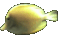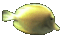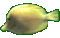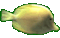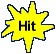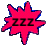Derivative Product Rule Practice with Power Rule     Math Help Fun     Game Tips:

- The derivative Product Rule is used on the product of two relations such as the product y=uv.
In general, the Product Rule states that the derivative of the product function y=uv  is    y'=uv'+u'v.

- For example, the unsimplified derivative of y=(x3x5)  is    y'=(x3)(5x4)+(3x2)(x5).

- This game uses the Product Rule   dy/dx = (u)(dv/dx) + (du/dx)(v)   and also the Power & Sum Rules.
Note that the symbols  dy/dx,  y' and  f '(x)  all represent the same derivative of y=f(x).

- The derivative Power Rule for y=xn yields the derivative  dy/dx = nxn-1.
For example, the derivatives of y=x4 and s(t)=t6 are respectively  dy/dx=4x3 and s'(t)=6t5.

- The Sum Rule states that the derivative of the sum function    y = g + h  is    y' = g' + h'.
For example, the derivative of    y = (x7 + x5)  is    y' = (7x6) + (5x4).

- Here is another example of the Product Rule y'=uv'+u'v :
The unsimplified derivative of y=(x3)(x7 + x5)  is    y'= (x3)(7x6 + 5x4) + (3x2)(x7 + x5).

- Your Game Score is reduced by the number of fish hits.

- To slow the game speed repeat tap/click on the word Slider.
- To increase the game speed repeat tap/click on the word Math.
- Speed can also be adjusted with a keyboard's - and + keys.

- Refresh/Reload the web page to restart the game.